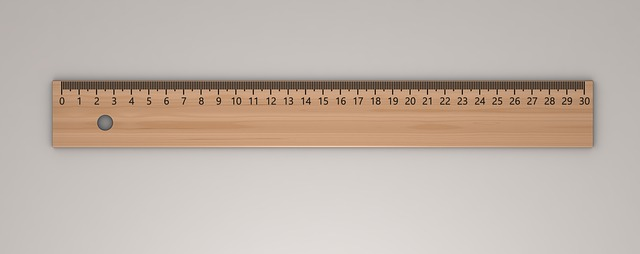# What is Infinity?Can you guess the Largest Number at the end of the following series?

0, 1, 2, 3, 4, 5, 6, 7, 8, 9, 10, 11, … , 100, 101,  … 1000, …,  9999999, 10000000, 10000001, 10000002, 10000003, ….. 999999999, 1000000000, 1000000001, 1000000002, ….

If you said, the largest number is (Infinity), your answer is wrong! There is no such “Number” called “ (Infinity)”. Infact,  is a symbol, which is used to represent the number series as “Endless” (i.e. Infinite or Boundless).

Let us keep the integers apart. Now, can you guess how many decimal numbers (Decimal Points or Real Numbers or Fractions) are there between the numbers 1 and 2?

Try writing out the series! You will find that, this will be an endless seriess too. Even if we say that, there are trillions of decimal points (or fractions or real numbers) between 1 and 2, our answer will be wrong! The right answer is that, there are infinite number of fractions or decimal points available between 1 and 2.

For instance, let us take a foot long ruler. We know that there are ten small line segments (millimeter) between 1st centimeter line segment and 2nd centimeter line segment. That is, 1.0, 1.1, 1.2, 1.3, …. 1.9, 2.0.If we divide these small segments  in to ten smaller segments each, we will get 1.00, 1.01, 1.02, 1.03, 1.04, … 1.09, 1.10, 1.11, 1.12, 1.13, … 1.19, 1.200, ………. 2.00. Each of these segments can further be divided in to 100, 1000, 10000, 100000, ………. and so on. There is no end to the infinitesimally smaller segments that we will obtain from this experiment. Such endless property of number sequence is represented by the symbol ∞ (Infinity).